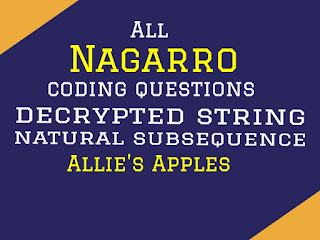# All coding questions and programs of today's Nagarro Coding RoundHello friends how are you , today in this blog i am going to teach, many important programs which is being asked by many companies , If you are preparing to join any company then this type of question will help you definitely.

I am writing this blog in December 2020 and today  theses question are  asked by a Popular Software company in their coding round question.

Question 1: Nth Character in Decrypted String

Every character in the input string is followed by its frequency . Write a function to decrypt the string the find the nth character of the decrypted string. If no character exists at that position then return -1.

Example :

If the string is "a3b2" then decrypted string will be "aaabb".

String:    a2b2c2
Number: 4
Output:   b
Explanation: The decrypted string of a2b2c2 will be aabbcc hence the 4th character in decrypted string is b.

Program :

``` class Dedecr_strptd {

public static String returnNthString(String str,int no)
{
String decr_str="";
char ch[]=str.toCharArray();
for (int i = 0; i < ch.length; i++) {

if(Character.isLetter(ch[i]))
{
decr_str=decr_str+ch[i];
}
else
{
for (int j = 1; j < Character.getNumericValue(ch[i]); j++) {
decr_str=decr_str+ch[i-1];
}
}

}
if(decr_str.length()<no)
return "-1";
else
return decr_str.charAt(no-1)+"";
}

public static void main(String[] args) {
System.out.println(returnNthString("a2b2c3",5));
}

}
/*
###Output###
c
*/
```

Question 2: Natural Subsequence

In Timesort we try to use the natural sorted subsequence already present in the array which is to be stored. The natural sorted sequences are those which are somehow already present in the sorted order in the provided array.

You task is to find the length of the longest natural sorted subsequence already present in the given string.

Example 1
String:    abzd
Output:   3
Explanation: abz or abd are the longest subsequences

Example 2
String:    bcdabdz
Output:   4
Explanation: bcdz or abdz are the longest subsequences

Program :
```class LIS
{
public static void main(String[] args)
{
String s="abzd";
char arr[]=s.toCharArray();
int max=0,mx,ele;
for(int i=0;i<arr.length;i++)
{
mx=0;
ele=arr[i];
for(int j=i;j<arr.length;j++)
{
if(ele<arr[j])
{
ele=arr[j];
mx++;
}
}
if(max<mx)
max=mx;
}
System.out.println("Length of Longest LIS is "+(max+1));
}
}
/*
###OUTPUT###
Length of Longest LIS is 3
*/
```

Question 3: Allie's Apples Program

I think there is no need of descripting of this question because this question is known by its name and it also very lengthy description of this program so i am just going to give the simplest code for this program.

Program :

```class Apple
{
{
double appleInSquare = 0;
int unit =0;
while(minmumApples>appleInSquare){
unit++;
appleInSquare +=12*Math.pow(unit,2);
}
return unit*8;
}

public static void main(String[] args)
{
}
}
/*
###OUTPUT###
8
8
16
*/
```

Request:-If you found this post helpful then let me know by your comment and share it with your friend.

If you want to ask a question or want to suggest then type your question or suggestion in comment box so that we could do something new for you all.

If you have not subscribed my website then please subscribe my website. Try to learn something new and teach something new to other.

Thanks.

Don't use my blog post without my permission.